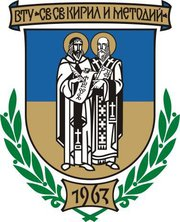Journal Pedagogical Almanac
“ST. CYRIL AND ST. METHODIUS” UNIVERSITY OF VELIKO TARNOVO - UNIVERSITY PRESS

## Solving a Triangle in Teaching Primary School Mathematics

Authors:
Ivanka Mincheva St. Cyril and St. Methodius University of Veliko Tarnovo, Bulgaria

Pages: 235-259
DOI: https://doi.org/10.54664/MBNJ2930

### Abstract:

The paper studies solving a triangle in primary school mathematics education. It proposes a system of problems reflecting the classification of the concept of triangle according to the sides and the angles. Each subsystem of a given main system includes a basic problem with generalized formulation and a sample solution followed by problems illustrating the basic problem. The methodological analysis encompasses some didactic components – short description, construction/drawing, sample solution, necessary component concepts, component pieces of knowledge and component problems. All drawings in the study have been made by using the mathematical software GeoGebra in order to ensure dynamism and clarity, and subsequently to achieve easier understanding of a problem and finding out its solution.

### Keywords:

GeoGebra; triangle; mathematics education; primary school; mathematical problem; system of problems; problem solution